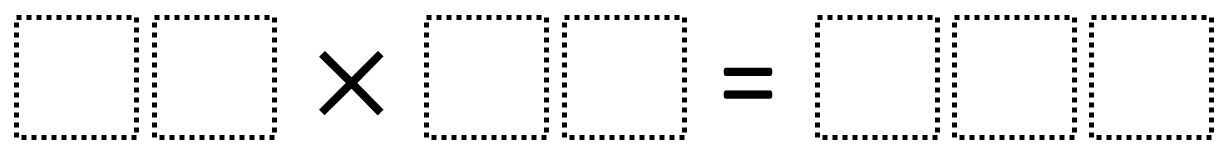# Multi-Digit Multiplication 1

Directions: Using the digits 0 to 9 at most one time each, place a digit in each box to create a true equation.### Hint

What two-digit numbers would be too low/high that when multiplied together would not make a three-digit product?

There are many possible true equations including 32 x 15 = 480 and 52 x 15 – 630.

Source: Robert Kaplinsky

## Equivalent Expressions with Fractions

Directions: Using the digits 0 to 9 at most one time each and choosing either …

1.2.3.